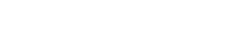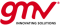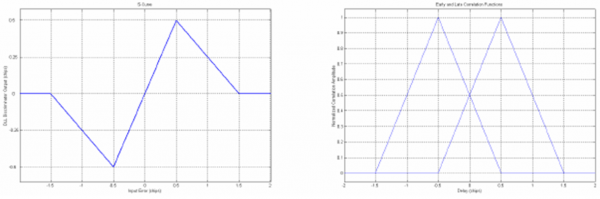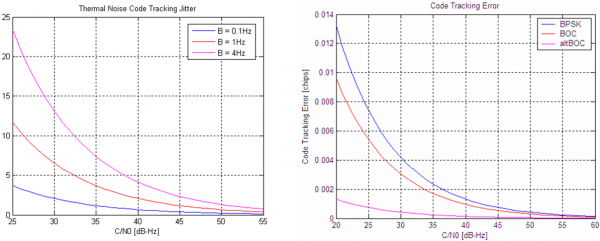If you wish to contribute or participate in the discussions about articles you are invited to contact the Editor

# Delay Lock Loop (DLL)Receivers
Title Delay Lock Loop (DLL)
Edited by GMV
Year of Publication 2011Delay Lock Loops are part of the receiver's signal tracking loops, and aim at tracking the code delay of the incoming GNSS signal. The DLL provides a correction of the current observed delay, and this correction is applied to the local replica code generators, in order to keep the local replica as "matched" as possible with the incloming signal.

## Principle

The Delay Lock Loop (DLL) tracks and estimates the current misalignment between the locally generated PRN code replica and the incoming signal, within the tracking loops. For that purpose, the DLL uses integrations, filters and Numerical Control Oscillators (NCO) – described here – as any other typical loop. In this case, the specificity relies on the discriminator used to assess the error of the current delay estimate.

The DLL actually uses two additional correlators: Early and Late, which are delayed and advanced replicas of the Prompt code. The difference between the Early and the Late correlators produces a so called S-curve. The DLL actually tracks the zero-crossing of this S-curve, in order to estimate the current error, which is then fed back to the local code generation block to correct the previous estimate of the incoming code delay. This principle is illustrated in Figure 1, where the Early and Late correlations are shown, as well as the S-curve produced by their difference.Figure 1: Early and Late correlation functions (right) and S-curve: E-L (left).

As shown here, the expressions for the Early and Late replicas are given by:

$\displaystyle{ I_E = Ad R_{x} (\tau_e-\frac{\delta}{2}) cos(\phi_e)\, }$

$\displaystyle{ Q_E = -AdR_{x}(\tau_e-\frac{\delta}{2})sin(\phi_e)\, }$

$\displaystyle{ I_L = Ad R_{x} (\tau_e+\frac{\delta}{2}) cos(\phi_e)\, }$

$\displaystyle{ Q_L = -AdR_{x}(\tau_e+\frac{\delta}{2})sin(\phi_e)\, }$

where:

• $\displaystyle{ \tau_e\, }$ is the error of the code delay estimated at the receiver.
• $\displaystyle{ \phi_e\, }$ is the error of the carrier phase estimated at the receiver.
• $\displaystyle{ E\, }$, $\displaystyle{ P\, }$, and $\displaystyle{ L\, }$ indices stand for Early, Prompt and Late, respectively.
• $\displaystyle{ \delta\, }$ is the Early-Late spacing.
• $\displaystyle{ A\, }$ is the amplitude.
• $\displaystyle{ d\, }$ is the navigation message.
• $\displaystyle{ R_x\, }$ is the autocorrelation function of the x PRN code.

## Discriminators

Two of the most commonly used discriminators are:

• Non-coherent Early minus Late Power (NELP), defined as:

$\displaystyle{ \frac{(I_E^2 + Q_E^2) - (I_L^2 + Q_L^2)}{2}\, }$

• Dot Product:

The quasi-coherent dot product power discriminator can be written as:

$\displaystyle{ \frac{[(I_E - I_L) I_P] + [(Q_E - Q_L) Q_P]}{2}\, }$

Whenever the PLL is locked, the following coherent dot product can be used, with lower computational burden:

$\displaystyle{ \frac{(I_E - I_L) I_P}{2}\, }$

• Normalized Early minus Late Envelope, given by:

$\displaystyle{ \frac{1}{2} \frac{(E - L)}{E + L}\, }$

where:

• $\displaystyle{ E= \sqrt{I_E^2+Q_E^2}\, }$ is the Early correlation power.
• $\displaystyle{ L= \sqrt{I_L^2+Q_L^2}\, }$ is the Late correlation power.

Normalized versions of the discriminators are often used, in order to remove amplitude sensitivity (especially useful in environments where the carrier to noise ratio changes rapidly).

## Performance

In optimal conditions, the main sources of errors in the DLL are thermal noise code jitter and dynamic stress error. Using techniques such as carrier aiding, the dynamic stress error of the DLL can be greatly reduced, leaving thermal noise as the main error source. The thermal noise code tracking jitter (in chips) for a non-coherent DLL discriminator can be approximated by:

$\displaystyle{ \sigma_{th}=\frac{1}{T_c} \sqrt {\frac{B_n \int_{-B_{fe}/2}^{B_{fe}/2} S_s(f)sin^2(\pi f \delta T_c)df}{(2 \pi)^2 C/N_0 [\int_{-B_{fe}/2}^{B_{fe}/2} f S_s(f)sin(\pi f \delta T_c)df]^2}} \times \sqrt { 1 +\frac {\int_{-B_{fe}/2}^{B_{fe}/2} S_s(f)cos^2(\pi f \delta T_c)df} { T C/N_0 [\int_{-B_{fe}/2}^{B_{fe}/2} S_s(f)cos(\pi f \delta T_c)df]^2}}\, }$

where:

• $\displaystyle{ T_c\, }$ is the chip period [s].
• $\displaystyle{ R_c\, }$ is the chipping rate [chip/s].
• $\displaystyle{ B_{fe}\, }$ is the double sided front-end bandwidth [Hz].
• $\displaystyle{ B_{n}\, }$ is the loop noise bandwidth [Hz].
• $\displaystyle{ S_s(f)\, }$ is the power spectral density of the signal, normalized to unit area over infinite bandwidth.
• $\displaystyle{ \delta\, }$ is the Early-Late spacing.

Figure 2 depicts the code tracking error (due to thermal noise) for different loop noise bandwidths but also different modulations, namely BPSK(1), BOC(1,1) and AltBOC (15,10).Figure 2: Impact of loop bandwidth (left) and modulation (right) on the DLL thermal noise jitter performance.

These results illustrate the fact that lower loop noise bandwidths (and longer integration times) lead to noise reduction, hence higher performances, as discussed here. The impact of the modulations is expressed by the Power Spectral Density (PSD), i.e. the spreader the signal (narrower correlation peaks), the higher performances can be reached.